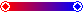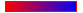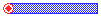property Bar.StartColor as Color
Returns or sets a value that indicates the color for the left side corner.Type Description Color A Color expression that indicates the color for the starting part of the bar.
Use the StartColor property to specify the color to fill the start part of the bar, if the StartShape property is not exShapeIconEmpty or Pattern is exPatternBox. Use the Color property to specify the color to fill the middle part of the bar. Use the EndColor and EndShape properties to define the appearance of the starting part of the bar. Use the AddShapeCorner property to add custom icons to the bars. In this case, the icon is processed before displaying based on the StartColor/ EndColor property. For instance, if you add an black and white icon, and the StartColor/EndColor is red, the icon will be painted in red. Instead, if the StartColor/EndColor property is -1 ( 0xFFFFFFFF, not white which is 0x00FFFFFF ), the icon is painted as it was added using the AddShapeCorner without any image processing. If the Pattern property is exPatternBox, the StartColor and EndColor properties defines the start and ending color to show a gradient bar.

The following VB sample changes the "Task" bar visual appearance using liner gradient with margins as shown:

```With G2antt1.Chart.Bars.Item("Task")
.Color = vbWhite
.Pattern = exPatternBox
.StartShape = exShapeIconCircleDot
.StartColor = vbRed
.EndShape = exShapeIconCircleDot
.EndColor = vbBlue
End With```

The following VB sample changes the "Task" bar visual appearance using liner gradient with solid border as shown:

```With G2antt1.Chart.Bars.Item("Task")
.Color = vbRed
.Pattern = exPatternBox
.StartColor = vbRed
.EndColor = vbBlue
End With```

The following VB sample defines a new bar that looks like this:

```With G2antt1.Chart.Bars.Add("Task2")
.Color = RGB(0, 0, 255)
.StartShape = exShapeIconCircleDot
.StartColor = RGB(255, 0, 0)
End With```

The following C++ sample defines a bar that looks like this above:

```CBar bar = m_g2antt.GetChart().GetBars().Add("Task2");
bar.SetColor( RGB(0, 0, 255) );
bar.SetStartShape( 4 /* exShapeIconCircleDot*/ );
bar.SetStartColor( RGB(255, 0, 0) );```

The following VB.NET sample defines a bar that looks like this above:

```With AxG2antt1.Chart.Bars.Add("Task2")
.Color = RGB(0, 0, 255)
.StartShape = EXG2ANTTLib.ShapeCornerEnum.exShapeIconCircleDot
.StartColor = RGB(255, 0, 0)
End With```

The following C# sample defines a bar that looks like this above:

```With AxG2antt1.Chart.Bars.Add("Task2")
.Color = RGB(0, 0, 255)
.StartShape = EXG2ANTTLib.ShapeCornerEnum.exShapeIconCircleDot
.StartColor = RGB(255, 0, 0)
End With```

The following VFP sample defines a bar that looks like this above:

```with thisform.G2antt1.Chart.Bars.Add("Task2")
.Color = RGB(0, 0, 255)
.StartShape = 4 && exShapeIconCircleDot
.StartColor = RGB(255, 0, 0)
EndWith```

In VB.NET or C# you require the following functions until the .NET framework will provide:

You can use the following VB.NET function:
```     Shared Function ToUInt32(ByVal c As Color) As UInt32
Dim i As Long
i = c.R
i = i + 256 * c.G
i = i + 256 * 256 * c.B
ToUInt32 = Convert.ToUInt32(i)
End Function ```
You can use the following C# function:
```private UInt32 ToUInt32(Color c)
{
long i;
i = c.R;
i = i + 256 * c.G;
i = i + 256 * 256 * c.B;
return Convert.ToUInt32(i);
}```